## Using Calculations

When using number fields, you are able to easily perform calculations. In this article, we will show you how to set up a calculation field.

Note : The Total fields cannot be used within calculations. Within this article, only Number fields are used.

Access the form editor by clicking on Forms, then on your desired form.

1. 1) First, you will need to add your Number fields. In this example, we will add 2 different number fields and name them First Number and Second Number.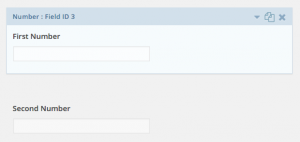2. 2) Next, a third Number field will be added which will handle the result of the calculation. For this example, we will name it Total.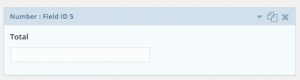3. 3) Within the Result field access the field settings by clicking on the field. Inside the field settings, you will notice a checkbox labeled Enable Calculation. Select this box to enable this field to be populated based on a calculation result.

Note: Ensure that this field is positioned after the Number fields that are being used as the basis for the calculation. If reversed, the calculation will not work correctly.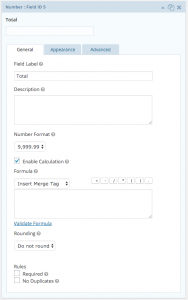4. 4) Once the Enable Calculation checkbox is selected, additional options will appear below it. This is where you will configure the fields to be calculated. You'll need to add the first field to be calculated. To do so, click on the dropdown labeled Insert Merge Tag, then and select your first field in the calculation.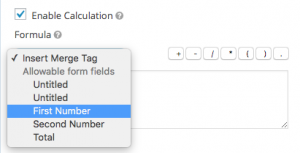5. 5) Then, based on how you want to calculate this field, use the symbol buttons to the right of the drop down to add, subtract, multiply, divide, etc. your second field.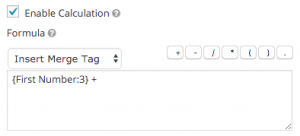6. 6) Of course, you will now need to add your next field. Jump back to the drop down and click on the next field in your calculation.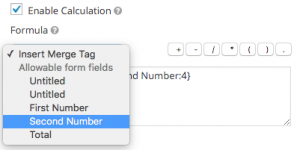7. 7) Now that your calculation is all set up, check it by clicking Validate Formula. If it says that your calculation is invalid, go back and take another look.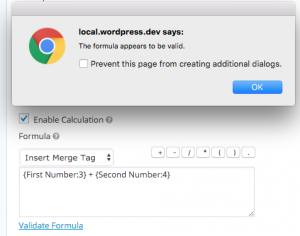Your calculation is all set. Be sure to save your form settings to ensure the field settings are valid.

Submit a request
From URJ.org
Join the Discussion

#### Community browse

Most Recent Articles
See more